Math Puzzles

# The Cookie Puzzle that’s driving people crazy!

497 views

 1 Introduction 2 Cookie Puzzle 3 Conclusion 4 FAQs 5 External References

## Introduction

Did you know that 4th December is celebrated as National Cookie day? As odd as the day sounds, we at the Cuemath wouldn’t leave a chance to put an interesting mathematical twist to the day. As a result of which this very interesting puzzle was formed: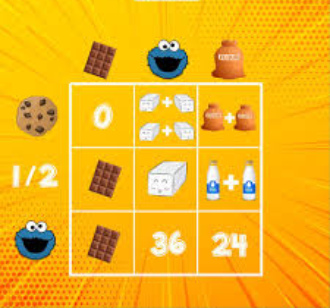Can you find out the values of the elements, namely the cookie, the cookie monster, the sugar cubes, flour bags, and the milk carton?

So what’s the solution?

The puzzle works in a grid multiplication format, meaning- you need to multiply each element on the outside of the grid to get the value inside the grid.

Let’s consider each column as Column A, B, and C.
And each row as Row 1, 2, and 3.
So to get the value of the first box, you will have to multiply the element on top of column A with the element beside Row 1.

Let’s consider column A:

Write all the equations you can form from the multiplication of the rows with column A.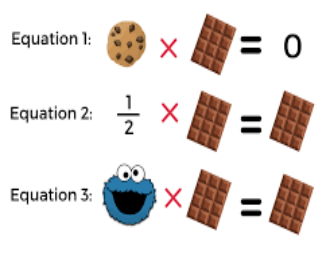We can derive from the first equation that the value of either the chocolate or the cookie is 0 (Anything multiplied by 0, will give 0 as a product). But the next two equations make it clear that it’s the chocolate that has a value of 0.

Let’s consider column B now:

Write all the equations from the multiplication of the rows with column B.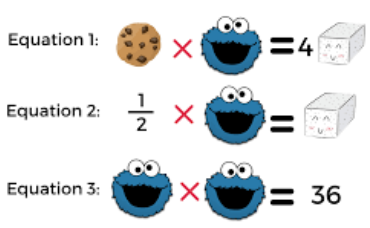The easiest way to solve this is to start from the last equation:

Therefore, Cookie Monster = √36 = 6

Now, use the value of Cookie Monster in the second equation:

½ x Cookie Monster = Sugar Cube

I.e ½ x 6 = Sugar Cube

Therefore, Sugar Cube = 3.

Using the value of the cookie monster and Sugar Cube in the first equation you’ll get:

I.e Cookie = 12/6 = 2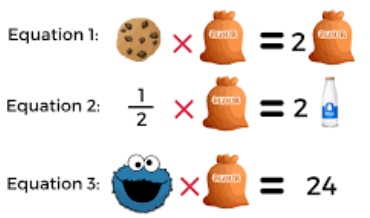Consider Column C:

Since we already know the value of the cookie monster, we’ll start solving equation 3.

Cookie Monster x Flour bag = 24

I.e  6 x Flour bag = 24

Therefore, Flour bag = 24/6 = 4

Substituting the value of flour bag into the second equation we have:

½ x Flour bag = 2 Milk Bottles

I.e ½ x 4 = 2 Milk Bottles

Therefore each milk bottle = 1

To sum it all up, the values of the elements in the grid are:

Did you get it right?

Why should your child learn multiplication using a multiplication grid?

The multiplication grid method acts as a great starting point to help your child understand and solve multiplicative problems involving multi-digit numbers. We’ve often seen, kids struggle with multi-digit multiplication, as they’re unable to break the numbers into smaller chunks.

The grid method, although different from how your child would learn multiplication, is often considered to be more reliable as children make less mistakes when using the grid.

This method of multiplication greatly emphasizes on the application of repeated addition.

For example – to multiply big numbers, like 234 with 32 your child may take a long time, or be perplexed by the size of the numbers. However, they can easily solve the same using their multiplication table knowledge of 2, 3 and 4. Here’s how: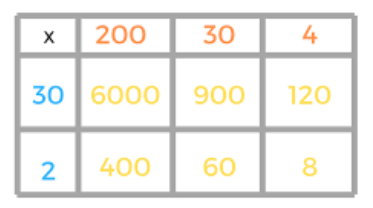Upon adding all the values inside the grid children are able to reach the multiplicative value of 234 x 32= 7488, just by knowing their 2,3 and 4’s tables.

The traditional method is although ultimately faster and much more compact; it requires two significantly more difficult multiplications that children may at first struggle with. Compared to the grid method, traditional long multiplication may also be more abstract and involves less clarity, so some children find it harder to remember what is to be done at each stage and why.

The grid method can also be used not just to multiply general numbers, but also to multiply fractions and decimal numbers as well.

At Cuemath, we teach children to think about more than one way of solving math problems. Also, a Cuemath student gains an advantage in competitive exams as they are trained to use visual problem-solving methods, which yield results in a much shorter period of time.

Also, try our exclusive Puzzle Time series: Puzzle Time

If you ever want to read it again as many times as you want, here is a downloadable PDF to explore more.

Cuemath students enjoy Cuemath’s Puzzle Cards that are curated keeping in mind that age is no bar to enjoy them!

## Conclusion

Innovation leads to new products and processes that sustain our economy. This innovation and science literacy depends on a solid knowledge base in the STEM areas. It is clear that most jobs of the future will require a basic understanding of math and science.Science, technology, engineering and mathematics workers play a key role in the sustained growth and stability of the world economy and are a critical component to helping the world win the future.

STEM education creates critical thinkers, increases science literacy, and enables the next generation of innovators.

Cuemath, a student-friendly mathematics and coding platform, conducts regular Online Live Classes for academics and skill-development and their Mental Math App, on both iOS and Android, is a one-stop solution for kids to develop multiple skills. You can book a Free Class here and know more about the pricing and fees from the Cuemath fee for all grades.